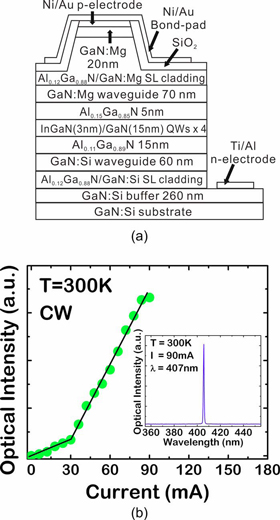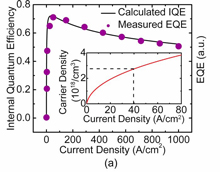This Site Web

 FREE subscription Subscribe for free to receive each issue of Semiconductor Today magazine and weekly news brief.

# News

## 3 December 2009

### Auger largely responsible for limited LED efficiency

Many researchers blame Auger recombination for the observed drops in efficiency of indium gallium nitride (InGaN) LEDs at high drive currents (above current densities in the range 10-50A/cm2). This limits the prospects for replacing traditional lighting with more efficient LEDs at low cost.

University of Michigan researchers have used large signal modulation measurements on InGaN lasers to derive the Auger coefficient for InGaN material at room temperature [Zhang et al, Appl. Phys. Lett., vol95, p201108, 2009]. The value that is obtained compares well with that obtained from considering the ‘efficiency droop’ in LEDs with a similar wavelength as deriving from the Auger mechanism, leading the researchers to write: “It is apparent that Auger recombination is largely responsible for limiting device efficiencies at high injection currents.”LEDs and laser diodes depend on bringing together negatively and positively charged carriers (‘electrons’ and ‘holes’, respectively) in semiconductor materials to recombine, releasing energy in the form of photons/light. Auger recombination refers to a competing process where electrons and holes recombine, but the energy released is transferred to another electron or hole. This competing mechanism is expected to increase with charge carrier densities, and hence at high drive currents.

Figure 1: Schematic of InGaN/GaN MQW laser heterostructure (a) and measured light-current characteristics (b).

The Michigan group used a multi-quantum well (MQW) laser (Figure 1) with InGaN wells and GaN barriers that emitted at a wavelength around 407nm (near ultraviolet). The Auger coefficient was derived by measuring the turn-on delay for stimulated emission and comparing the behavior with injected current with what is expected from an analysis using the carrier rate equation below threshold. The recombination was modeled up to terms cubic in the charge carrier density. The linear and quadratic terms were taken as representing the Shockley–Read–Hall recombination rate (i.e. non-radiative recombination through intermediate levels in the energy bandgap) and the radiative recombination rate. The values of these other coefficients were derived from spectroscopy of the bandgap levels and a nitride device simulator, respectively. The turn-on delay was measured using 120 nanosecond pulses (15% duty cycle) from zero to a current above threshold. The rise time was 100ps (20%-80%). The Auger coefficient is given by the Michigan group as being Ca = 1.5x10−30cm6/sec at 300K.Figure 2: Michigan’s calculated internal quantum efficiency (solid line) as a function of injection current of near-UV InGaN/GaN QW LED, based on measured Auger recombination coefficient. Solid circles show reported data [Yang et al, IEEE Trans. Electron Devices, vol55, p1771, 2008] for an LED emitting at nearly the same wavelength. Inset: calculated relation between carrier density in the QWs and current density. The dashed lines signify the point of maximum efficiency.

This Auger coefficient was used to predict the internal quantum efficiency as a function of injected current density for 410nm (near-UV) InGaN LEDs. It was assumed that the diffusion length of the holes was long enough for a simple assumption of uniform hole density over the 12nm MQW structure (this is often not the case with InGaN MQWs). It was further assumed that all recombination occurs in the MQW region. A 70% internal quantum efficiency peak is predicted at ~40A/cm2. The predictions were compared with experimental results (Figure 2) given by West Virginia University [Yang et al, IEEE Trans. Electron Devices, vol55, p1771, 2008].

The Michigan group comments on the apparent inconsistency where the Auger coefficient is an order of magnitude lower than that in longer-wavelength indium gallium arsenide on indium phosphide (InGaAs/InP) MQWs, but where no efficiency droop is seen at comparable current levels. Michigan makes the point that carrier densities — the important factor in Auger recombination — are also affected by the thickness of the MQW region and the carrier lifetimes. For nitride devices the MQW is thinner and carrier lifetimes are longer, both factors increasing carrier densities for a given current density.

See related items:

InGaN LED spillover and efficiency droop

Fathoming efficiency droop in InGaN MQW-LEDs

See article: Solutions don’t solve droop controversy

Visit: www.eecs.umich.edu

The author Mike Cooke is a freelance technology journalist who has worked in the semiconductor and advanced technology sectors since 1997.